Topics: AI | Data Science | Data Visualization | Deep Learning | Machine Learning | NLP | Python | R | Statistics

KDnuggets Home » News » 2018 » Apr » Tutorials, Overviews » Swiftapply  – Automatically efficient pandas apply operations ( 18:n18 )

# Swiftapply  – Automatically efficient pandas apply operations

Tags: ,

Using Swiftapply, easily apply any function to a pandas dataframe in the fastest available manner.comments

By Jason Carpenter, University of San Francisco

Time is precious. There is absolutely no reason to be wasting it waiting for your function to be applied to your pandas series (1 column) or dataframe (>1 columns). Don’t get me wrong, pandas is an amazing tool for python users, and a majority of the time pandas operations are very quick.

Here, I wish to take the pandas apply function under close inspection. This function is incredibly useful, because it lets you easily apply any function that you’ve specified to your pandas series or dataframe. But there is a cost — the apply function essentially acts as a for loop, and a slow one at that. This means that the apply function is a linear operation, processing your function at O(n) complexity.

Experienced users of pandas and python may be well aware of the options available to increase the speed of their transformations: vectorize your function, compile it with cython or numba, or use a parallel processing library such as dask or multiprocessing. But there is likely a broad category of python users who are either unaware of these options, don’t know how to use them, or don’t want to take the time to add the appropriate function calls to speed up their operations.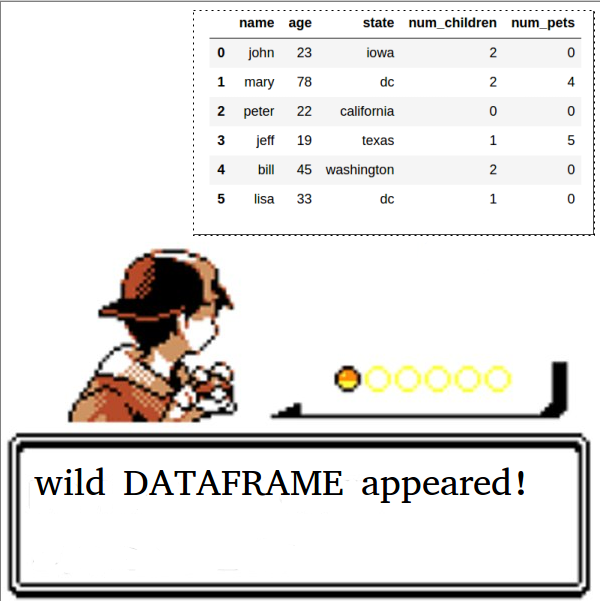What do you do?

### swiftapply

It’s highly effective!

Swiftapply, available on pip from the swifter package, makes it easy to apply any function to your pandas series or dataframe in the fastest available manner.

What does this mean? First, swiftapply tries to run your operation in a vectorized fashion. Failing that, it automatically decides whether it is faster to perform dask parallel processing or use a simple pandas apply.

So, how do we use it? First, let’s install swifter at the command line.

`\$ pip install swifter`

Next, import the function into your python notebook or .py file.

`from swifter import swiftapply`

Now, you are ready to use swiftapply.

`myDF['outCol'] = swiftapply(myDF['inCol'], anyfunction)`

### Examples

This notebook gives a couple examples of swiftapply usage on a >71 million rows SF Bay Area Bikeshare data set, but I will also provide examples inline here. All applied functions are in bold.

Example 1 (vectorized):

```def bikes_proportion(x, max_x):
return x * 1.0 / max_x

data['bike_prop'] = swiftapply(data['bikes_available'],
bikes_proportion,
max_x=np.max(data['bikes_available']))```

Example 2 (tries vectorized -> fails -> uses dask parallel processing instead):

```def convert_to_human(datetime):
return datetime.weekday_name + ', the ' + str(datetime.day) + 'th day of ' + datetime.strftime("%B") + ', ' + str(datetime.year)

convert_to_human)```

Example 3 (how to make non-vectorized code (13.8s) into vectorized code (231ms)):

```# Parallel processing b/c if-else statement makes it non-vectorized
def gt_5_bikes(x):
if x > 5:
return True
else:
return False

# computes in 13.8s
data['gt_5_bikes'] = swiftapply(data['bikes_available'], gt_5_bikes)

# Vectorized version
def gt_5_bikes_vectorized(x):
return np.where(x > 5, True, False)

# computes in 231ms
data['gt_5_bikes_vec'] = swiftapply(data['bikes_available'],
gt_5_bikes_vectorized)```

### Benchmarks

This notebook contains benchmarks using 4 different functions on the same >71 million rows data set.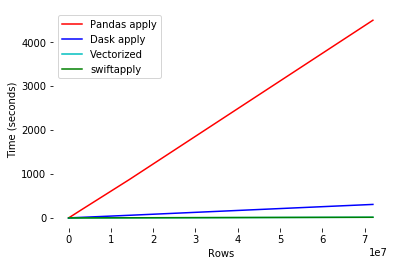Swiftapply vectorizes when possible for ≥100x speed increase

The first benchmark I will discuss is the pd.to_datetime function. Looking at the figures above (time in seconds v. number of rows), and below (log10 of both quantities), it becomes clear that using a pandas apply of pd.to_datetime is an incredibly slow operation (> 1 hour) on a data set of this size. Instead, it would be better to use the vectorized form of the operation, since it is a vectorized function. Swiftapply automatically does this, when possible.

```df['date'].apply(pd.to_datetime) # very slow

pd.to_datetime(df['date']) # vectorized - very fast

swiftapply(df['date'], pd.to_datetime) # also vectorized - very fast```

Below, I’ve included the log10-log10 plot of time (seconds) v. rows so that we can interpret the measurable difference in performance. Remember, this means that every tickmark represents a 10x change in the value. That means that the difference between pandas and dask is 10x, and the difference between pandas and swiftapply/vectorized is 100x.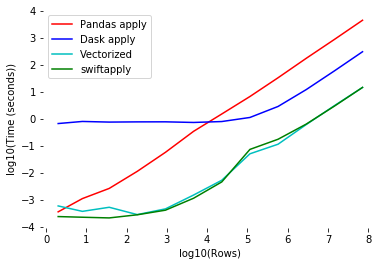X is 1, 10, 100, 1000, …

In the event that you wish to apply a function that is not vectorizable, like convert_to_human(datetime) function in example 2, then a choice must be made. Should we use parallel processing (which has some overhead), or a simple pandas apply (which only utilizes 1 CPU, but has no overhead)?

Looking at the below figure (log10 scale), we can see that in these situations, swiftapply uses pandas apply when it is faster (smaller data sets), and converges to dask parallel processing when that is faster (large data sets). In this manner, the user doesn’t have to think about which method to use, regardless of size of the data set.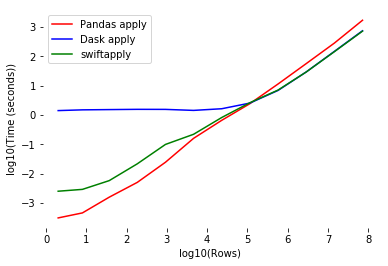Swiftapply converges to pandas apply on small datasets and dask parallel processing on large ones

Admittedly, the difference between swiftapply/dask and pandas doesn’t look very impressive in the above plot when the number of rows is high (log10 rows > 5). However, when we convert it to normal scale below, we see the true performance gain. Even with this slow non-vectorizable function, swiftapply’s utilization of dask parallel processing increases speed by 3x.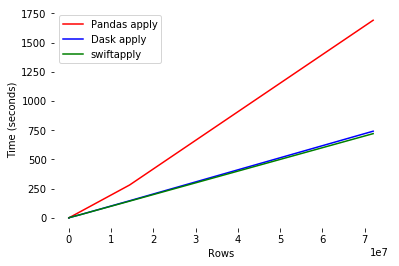This adaptive functionality makes swiftapply an efficient, easy to use apply function for all situations.

If you wish to use or contribute to the package, here is the github repository: https://github.com/jmcarpenter2/swifter

Bio: Jason Carpenter is a Master's Candidate in Data Science at University of San Francisco, and a Machine Learning Engineer Intern at Manifold.

Original. Reposted with permission.

Related:

Top Stories Past 30 Days
Most SharedGet KDnuggets, a leading newsletter on AI, Data Science, and Machine Learning